# 集支付函数的向量均衡问题存在性定理Existence Theorems of Vector Equilibrium Problems with Set Payoffs Functions

DOI: 10.12677/PM.2020.104037, PDF, HTML, XML, 下载: 155  浏览: 412  国家自然科学基金支持

Abstract: Based on the definitions of cone semicontinuous and cone quasiconvex of payoff function, the existence theorems of solutions for vector equilibrium problems with payoff function are obtained under new assumptions by using the classical Fan-KKM theorem and separation theorem. Finally, the feasibility of the results is verified by an example.

1. 引言

$F\left(\stackrel{¯}{x},y\right)\not\subset -S\\left\{\theta \right\}$ , $\forall y\in {X}_{0}$

2. 预备知识

S的对偶锥 ${S}^{\text{*}}:=\left\{{s}^{\text{*}}\in {V}^{*}:{s}^{\text{*}}\left(s\right)\ge 0,\forall s\in S\right\}$

$F\left(\stackrel{¯}{x},y\right)\not\subset -S\\left\{\theta \right\}$ , $\forall y\in {X}_{0}$

$F\left({x}_{0}\right)\cap \left(b+S\right)=\varnothing \text{ }\left(F\left({x}_{0}\right)\cap \left(b-S\right)=\varnothing \right)$

$\exists {x}_{0}$ 的邻域U，s.t.，

$F\left(x\right)\cap \left(b+S\right)=\varnothing \text{ }\left(F\left(x\right)\cap \left(b-S\right)=\varnothing \right)$ , $\forall x\in U$

$F\left(x\right)=\left\{\begin{array}{l}\left[-1,1\right],\text{\hspace{0.17em}}\text{\hspace{0.17em}}x=0\\ 0,\text{ }\text{ }\text{\hspace{0.17em}}\text{ }\text{ }x\ne 0\end{array}$

$b>1$ ，有 $F\left(0\right)\cap \left(b+S\right)=\left[-1,1\right]\cap \left(b+S\right)=\varnothing$ 。由F的定义可知，一定存在0的邻域U，s.t.， $F\left(x\right)\cap \left(b+S\right)=\varnothing$$\forall x\in U$ 。即F在0点 ${R}_{+}$ -拟上半连续。

$b=-\frac{1}{2}$$b+S=\left\{x|x\ge -\frac{1}{2}\right\}$ 。显然， $F\left(0\right)=\left[-1,1\right]\not\subset b+S$ ，对0的任意邻域U， $F\left(x\right)\subset b+S$ 。显然，F在0点不满足文献  中相应的概念。

$le{v}_{F}\left(b\right):=\left\{x\in X:F\left(x\right)\cap \left(b+S\right)\ne \varnothing \right\}$

1) 对 $\forall {x}_{1},{x}_{2}\in {X}_{0}$ ，并且 $l\in \left[0,1\right]$ ，有

$F\left(l{x}_{1}+\left(1-l\right){x}_{2}\right)\subset F\left({x}_{1}\right)-S$$F\left(l{x}_{1}+\left(1-l\right){x}_{2}\right)\subset F\left({x}_{2}\right)-S$ ,

2) 对 $\forall {x}_{1},{x}_{2}\in {X}_{0}$ ，并且 $l\in \left[0,1\right]$ ，有

$F\left(l{x}_{1}+\left(1-l\right){x}_{2}\right)\subset co\left\{F\left({x}_{1}\right),F\left({x}_{2}\right)\right\}-S$ ,

${\xi }_{ka}=\mathrm{min}\left\{t\in R:z\in a+tk-S\right\}$ .

1) ${\xi }_{ka}\left(z\right)\le r⇔z\in a+rk-S$

2) ${\xi }_{ka}\left(z\right)\ge r⇔z\notin a+rk-\mathrm{int}S$

3) 如果 ${z}_{1}-{z}_{2}\in S⇒{\xi }_{ka}\left({z}_{1}\right)\ge {\xi }_{ka}\left({z}_{2}\right)$ ，那么 ${\xi }_{ka}\left(\cdot \right)$ 是单调递增的函数；

4) 若 ${z}_{1}-{z}_{2}\in \mathrm{int}S⇒{\xi }_{ka}\left({z}_{1}\right)>{\xi }_{ka}\left({z}_{2}\right)$ ，则 ${\xi }_{ka}\left(\cdot \right)$ 是严格单调递增的函数；

5) ${\xi }_{ka}\left(\cdot \right)$ 是连续的函数。

3. 主要结论

1) 对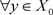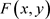是X上的S-拟上半连续，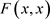是S-拟下半连续；

2) 对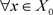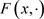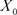上的真S-拟凸；

3)s.t.(3.1)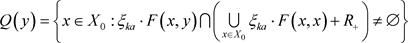,1) 对，都有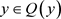，即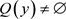2) 又因为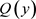为一个紧集，，且是紧的，故仅需证为闭集即可。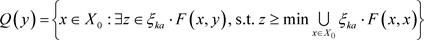,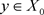3) 下面证明为一个KKM映象。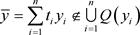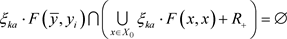,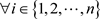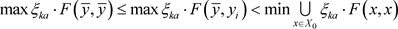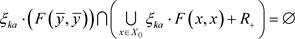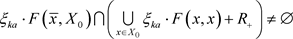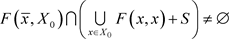(3.2)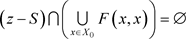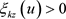,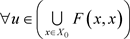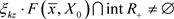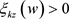,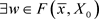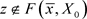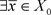, s.t.,,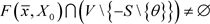i.e.,, s.t.,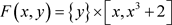1) 对是X上的S-拟上半连续，是S-拟下半连续；

2) 对上的自然S-拟凸；

3)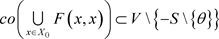s.t.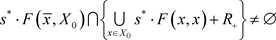(3.3)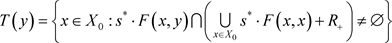,1) 对，都有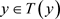，即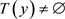2) 又因为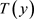为一个紧集，，且是紧的，故仅需证为闭集即可。,3) 下面证明为一个KKM映象。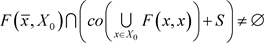,(3.4)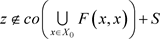,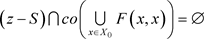,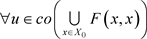,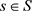(3.5)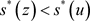,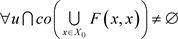s.t.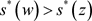,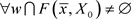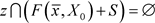(3.4)得证。

NOTES

*通讯作者。

  Bigi, G., Cap?t?, A. and Kassay, G. (2012) Existence Results for Strong Vector Equilibrium Problems and Their Applications. Optimization, 567-583. https://doi.org/10.1080/02331934.2010.528761  Chen, T., Zou, S.F. and Zhang. Y. (2019) New Existence Theorems for Vector Equilibrium Problems with Set-Valued Mappings. Journal of Nonlinear Functional Analysis, 2019, Article ID: 45. https://doi.org/10.23952/jnfa.2019.45  Lin, Y.C. (2009) On Generalized Vector Equilibrium Problems. Nonlinear Analysis, 70, 1040-1048. https://doi.org/10.1016/j.na.2008.01.030  Gong. X.H. (2001) Efficiency and Heing Efficiency for Vector Equilibrium Problems. Journal of Optimization Theory and Applications, 108, 139-154. https://doi.org/10.1023/A:1026418122905  Kien, B.T., Wong, N.C. and Yao, J.C. (2008) Generalized Vector Variational Inequalities with Star-Pseudomonotone and Discontinuous Operators. Nonlinear Analysis, 68, 2859-2871. https://doi.org/10.1016/j.na.2007.02.032  Zhang, Y., Li, S.J. and Li. M.H. (2012) Minimax Inequalities for Set-Valued Mappings. Positivity, 16, 751-770. https://doi.org/10.1007/s11117-011-0144-6  Han, Y. and Huang. N.J. (2018) Existence and Connectedness of Solutions for Generalized Vector Quasiequilibrium Problems. Journal of Optimization Theory and Applications, 179, 65-85. https://doi.org/10.1007/s10957-016-1032-9  Li, S.J., Chen, G. and Yang. X.Q. (2003) Generalized Minimax Inequalities for Set-Valued Mappings. Journal of Mathematical Analysis and Applications, 281, 707-723. https://doi.org/10.1016/S0022-247X(03)00197-5  Zhang, Y. and Li, S.J. (2014) Generalized Ky Fan Minimax Inequalities for Set-Valued Mappings. Fixed Point Theory, 15, 609-622.  张宇. 集值极大极小定理与集值博弈问题[M]. 31版. 北京: 科学出版社, 2018.  Fan, KY. (1961) A Generalization of Tychonoff’s Fixed Point Theorem. Mathematische Annalen, 142, 303-310. https://doi.org/10.1007/BF01353421  Gerstewitz, C. (1986) Nichtkonvexe trennungss?tze und deren anwendung in der theorie der vektoroptimierung. Seminarberichteder Secktion Mathematik der Humboldt-Universit?t zu Berlin, 19-31.  Certh, C. and Weidner, P. (1990) Nonconvex Separation Theorems and Some Applications in Vector Optimization. Journal of Optimization Theory and Applications, 67, 297-320. https://doi.org/10.1007/BF00940478